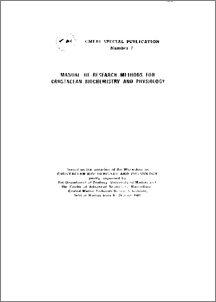# Regression (y=a+bx)

Paulraj, S and Mullainadhan, P (1981) Regression (y=a+bx). CMFRI Special Publication (7). p. 161.Preview
PDF
Spl_7-24.pdf

Download (147kB)
Related URLs:

## Abstract

In regression, the relationship of one variable with another is estimaed by expressing the one in terms of the linear function of the other. It is different from correlation (r) in that, in correlation the degree to which the two variables vary together is estimated. In both regression and correlation the values are continuous. The functional relationship in regression is a mathematical relationship which enables to predict the value of a variable y which corresponds to a given variable x. The relationship is determined by y=a+bx in which y is the function of x and is called the dependent variable, x the independent variable. By this formula when the independent variable (x) equals zero, the dependent variable equals' a'. This point is the intersection of the function line with the y axis which is called as ' y-intercept', and * b ' refers to the slope or the gradient of the function y=a+bx. ' b ' is called the regression coefficient and the formula is referred to as regression equation (Sokal & Rohlf, 1973).

Item Type: Article Regression (y=a+bx); Crustacean Biochemistry and Physiology Crustacean FisheriesFish and Fisheries > Biochemical Study ContributorsCMFRI-Kochi > Marine Capture > Crustacean FisheriesSubject Area > CMFRI > CMFRI-Kochi > Marine Capture > Crustacean FisheriesCMFRI-Kochi > Marine Capture > Crustacean FisheriesSubject Area > CMFRI-Kochi > Marine Capture > Crustacean Fisheries Geetha P Mrs 24 Sep 2010 06:10 09 Sep 2015 15:21 http://eprints.cmfri.org.in/id/eprint/3317

### Actions (login required)View Item